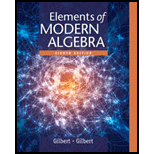# A permutation matrix is a matrix that can be obtained from an identity matrix I n by interchanging the rows one or more times (that is, by permuting the rows). For n = 3 the permutation matrices are I 3 and the five matrices. (Sec. 3.3 , # 22 c , 32 c , Sec. 3.4 , # 5 , Sec. 4.2 , # 6 ) P 1 = [ 1 0 0 0 0 1 0 1 0 ] P 2 = [ 0 1 0 1 0 0 0 0 1 ] P 3 = [ 0 1 0 0 0 1 1 0 0 ] P 4 = [ 0 0 1 0 1 0 1 0 0 ] P 5 = [ 0 0 1 1 0 0 0 1 0 ] Given that G = { I 3 , P 1 , P 2 , P 3 , P 4 , P 5 } is a group of order 6 with respect to matrix multiplication, write out a multiplication table for G . Sec. 3.3 , # 22 c , 32 c ≪ 22. Find the center Z ( G ) for each of the following groups G . c . G = { I 3 , P 1 , P 2 , P 3 , P 4 , P 5 } in Exercise 35 of section 3.1. 32. Find the centralizer for each element a in each of the following groups. c. G = { I 3 , P 1 , P 2 , P 3 , P 4 , P 5 } in Exercise 35 of section 3.1 Sec. 3.4 , # 5 ≪ 5. The elements of the multiplicative group G of 3 × 3 permutation matrices are given in Exercise 35 of section 3.1 . Find the order of each element of the group. Sec. 4.2 , # 6 ≪ 6. Let G be the group of permutations matrices { I 3 , P 1 , P 2 , P 3 , P 4 , P 5 } as given in Exercise 35 of Section 3.1 .### Elements Of Modern Algebra

8th Edition
Gilbert + 2 others
Publisher: Cengage Learning,
ISBN: 9781285463230### Elements Of Modern Algebra

8th Edition
Gilbert + 2 others
Publisher: Cengage Learning,
ISBN: 9781285463230

#### Solutions

Chapter
Section
Chapter 3.1, Problem 35E
Textbook Problem

## Expert Solution

### Want to see the full answer?

Check out a sample textbook solution.See solution

### Want to see this answer and more?

Experts are waiting 24/7 to provide step-by-step solutions in as fast as 30 minutes!*

See Solution

*Response times vary by subject and question complexity. Median response time is 34 minutes and may be longer for new subjects.